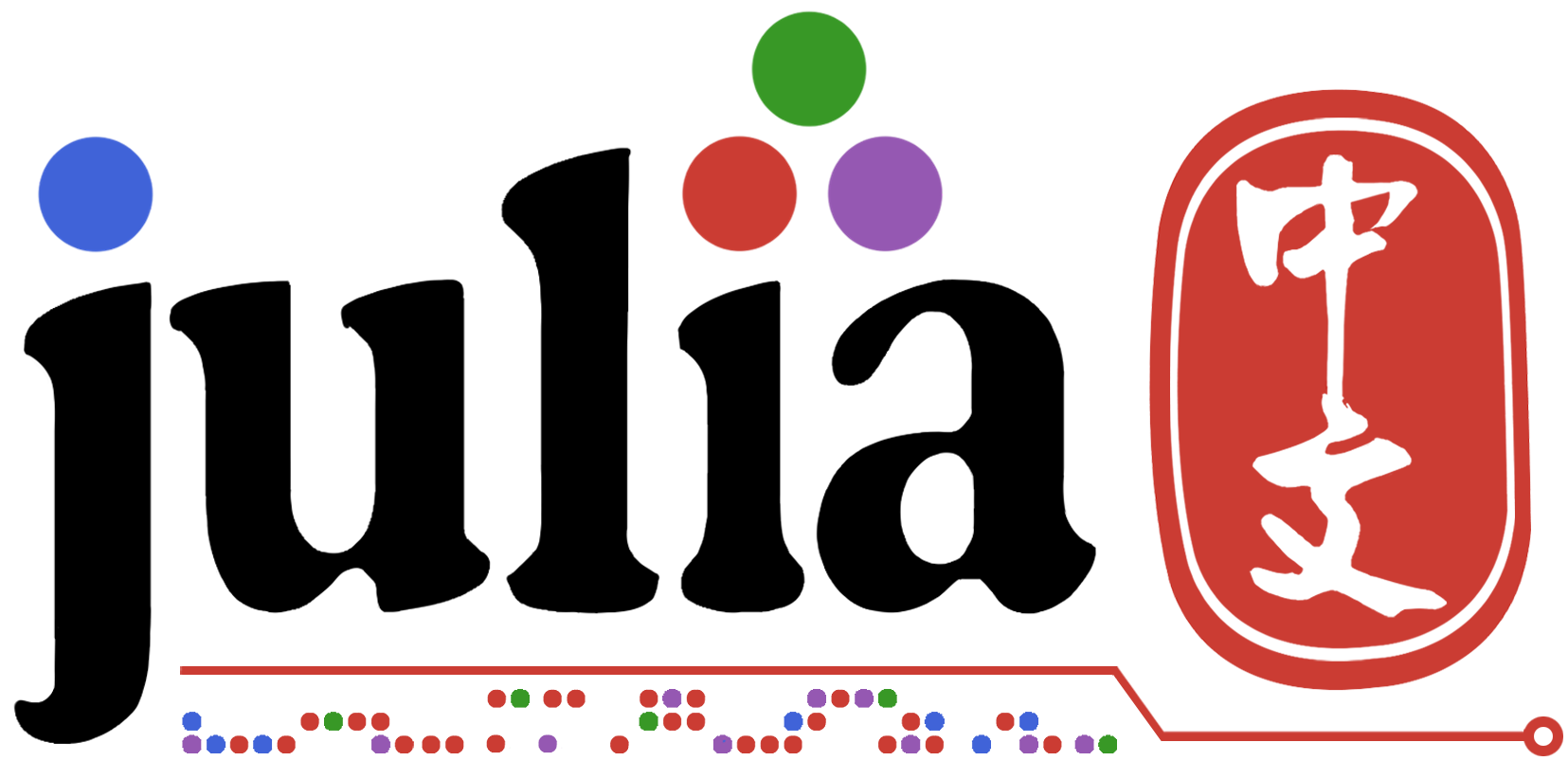6

# 线路Equations.jl

using LinearEquations sol = @linsolve x y begin x + y == 3 2x + 5y == 9 end sol # [x,y] = [2.0,1.0]

x, y = @linsolve x y begin 3(x - 2y) + 10 == y - 7 6x + 5y == 4 end x # -1.0 y # 2.0

x, y = @linsolve x y begin x + 2y == 2 -2//3*x + y == 1//2 end x # 3//7 y # 11//14

## 使用矢量变量

x, y = @linsolve x=>3 y=>3 begin 2eye(3)*x == y x == x x + x + x == 0.0 y[end] == 0.1 end x # [-0.1,0.05,0.05] y # [-0.2,0.1,0.1]

# 内部でやっていること

プログラム内でやっていることの本質は超単純です．未知変数を全て順番通りにxxベクトルに並べて，係数行列AAと既知ベクトルbbを作り，と解いているだけです．ただこの方法の場合，未知変数の数をとすると，より小さいサイズの行列で解けるような場合にも，必ずの大きさの係数行列を作らなければならないため，場合によってはかなり計算速度に効いてきます．ここらへんは，今後単純な条件の場合は，小さいサイズで解くように改良したいなと思っています． `x = A \ b` `n` `n` `n×n` `A`

### Juliaって本当にいい言語！もっともっと広まって欲しいですね！

Edit request

Stock

6Researcher in Geomechanics

Follow

Why not register and get more from Qiita?